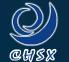### Product details

#### Supply Ability

• Warranty(Year)：1 Year

#### Product Description

* Characteristic: High in luminous effciency, less in power consumption, long in service life, white-golden in ray of light and strong in fogpenetrating capacity, Colour temperature 2K.
* Application: Suitable for lighting in the places of road, airport, wharf, station, factory and mine etc.
[td=1,1,11%]TYPE
[td=1,1,11%]Wattage
(W)
[td=1,1,11%]SON-T50[td=1,1,11%]50[td=1,1,11%]0.76[td=1,1,11%]85±10[td=1,1,11%]3600[td=1,1,11%]16000[td=1,1,11%]E27/E26[td=1,1,11%]38[td=1,1,12%]156[td=1,1,11%]SON-T70[td=1,1,11%]70[td=1,1,11%]0.98[td=1,1,11%]90±10[td=1,1,11%]6000[td=1,1,11%]16000[td=1,1,11%]E27/E26[td=1,1,11%]38[td=1,1,12%]156[td=1,1,11%]SON-T100[td=1,1,11%]100[td=1,1,11%]1.20[td=1,1,11%]90±10[td=1,1,11%]8500[td=1,1,11%]18000[td=1,1,11%]E27/E26[td=1,1,11%]38[td=1,1,12%]180[td=1,1,11%]SON-T150[td=1,1,11%]150[td=1,1,11%]1.80[td=1,1,11%]100±10[td=1,1,11%]16000[td=1,1,11%]24000[td=1,1,11%]E27/E26[td=1,1,11%]38[td=1,1,12%]180[td=1,1,11%]SON-T150[td=1,1,11%]150[td=1,1,11%]1.80[td=1,1,11%]100±10[td=1,1,11%]16000[td=1,1,11%]24000[td=1,1,11%]E40/E39[td=1,1,11%]48[td=1,1,12%]211[td=1,1,11%]SON-T250[td=1,1,11%]250[td=1,1,11%]3.00[td=1,1,11%]100±10[td=1,1,11%]26000[td=1,1,11%]24000[td=1,1,11%]E40/E39[td=1,1,11%]48[td=1,1,12%]257[td=1,1,11%]SON-T400[td=1,1,11%]400[td=1,1,11%]4.60[td=1,1,11%]100±10[td=1,1,11%]48000[td=1,1,11%]24000[td=1,1,11%]E40/E39[td=1,1,11%]48[td=1,1,12%]283[td=1,1,11%]SON-T600[td=1,1,11%]600
 OperatingCurrent (A) OperatingVoltage(V) Luminous(Lm) AverageLife(H) Base MaxDiameter (MM) MaxLength (MM) 6.20 110±10 70000 24000 E40/E39 48 310

### You May Like• Free Member